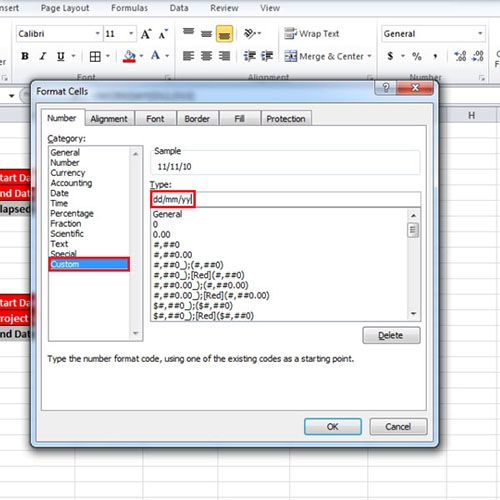## How to Calculate Days in Excel

Microsoft Excel is a detailed software that can be used as a multiple analysis tool. It gives simple options that can help you manage and manipulate data to derive desired or actual results. It is important to understand the workings of this software to be able to use it effectively. You can also calculate days in Excel to figure out the difference between two dates or to use a date and number of days to deduce the end date. To learn how to calculate number of days in Excel, follow this step by step tutorial.

Step # 1 – Calculate difference between dates

To learn how to calculate number of days in Excel, we will be working on two situations. In the first one, we have two dates and want to find the number of days elapsed between the two dates. Therefore, we will simply subtract the two cells and calculate the difference.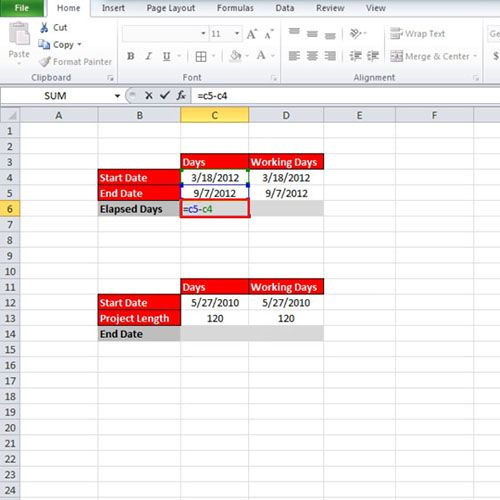Step # 2 – Use ‘NETWORKDAYS’ formula

If you want to exclude the weekend holidays from the result, write the following formula:

“=NETWORKDAYS(D4,D5)”

After using this function you will see that the number of days has decreased since weekends are excluded from the result now.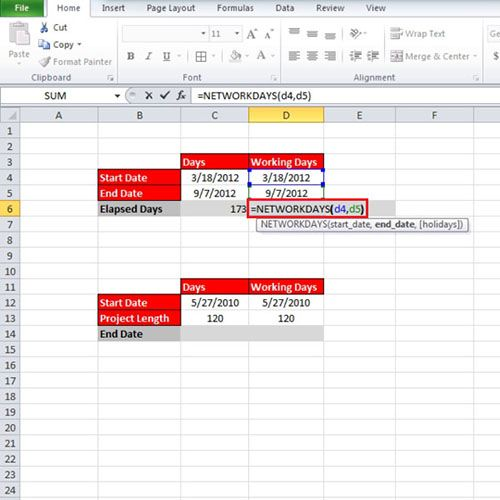Step # 3 – Calculate End date

To calculate days in Excel the previous formulas can be used but where we have a date and the total number of days, and we want to find out the end date, we will simply add 120 days to the ‘Start’ date. Use the following:

“=C12+C13”

With that done, we will get a date but again this date is calculated on the assumption that no holidays would come in between during these 120 days.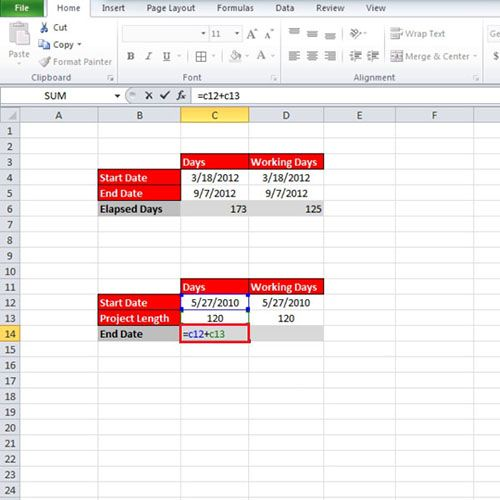Step # 4 – Use ‘WORKDAY’ formula

If you want to calculate the ‘End’ date keeping in consideration only the weekdays then, use the following formula:

“=WORKDAY(D12,D13)”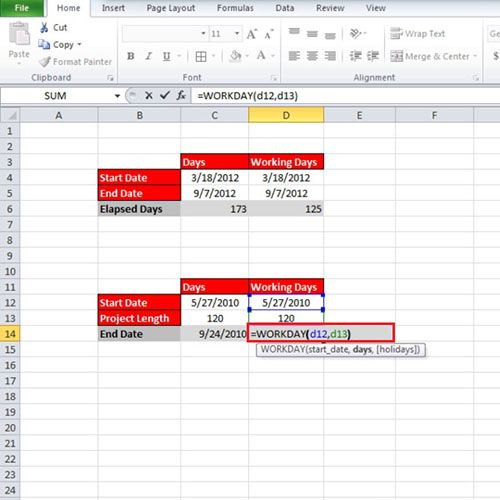Step # 5 – Change Cell format

Format the cell by right clicking and opening up the ‘Format Cells’ option. Click on “Customs” and write

“dd/mm/yy”

This is so that the output can be shown in a ‘Date’ format, with the day showing first, then the month and then the year.